##### The Japanese Bonsai specialist
Direct order Contact Help / Services Newsletter Pay in 3 times without charges, from 135 euros in your shopping cart (France residents only)# Japanese black rope +- 20 metres 'syuro nawa'

'shyuro nawa' (palm tree rope)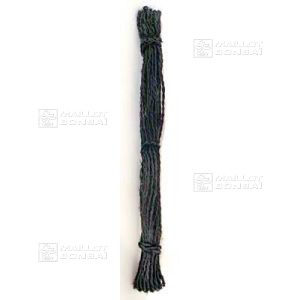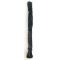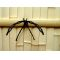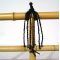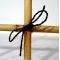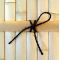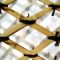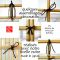ref. : 4312

5,35

Available quantity : 43Order

###### Description

1 spool, 20 metres of Japanese palm tree string for tying together traditional bamboo fences such as Kinkakuji-gaki and Koetsu-gaki. Ø +- 3 mm. The black rope’s other use is to fix bonsai to their supports so they don’t fall over in the wind. Soak the string in water for 20 minutes to soften the fibres for easy handling.

#japanese 3.8 #metres 3.6 #bonsai 3.6 #black 3.5 #rope 3.4 #string 2.6 #syuro 2.5 #tools 2.5 #gaki 2.4 #nawa 2.4

Formule
(( ROUND((CHAR_LENGTH(b.article_nom)-CHAR_LENGTH(REPLACE(b.article_nom, 'japanese', '')))/LENGTH('japanese')) + ROUND((CHAR_LENGTH(b.article_description)-CHAR_LENGTH(REPLACE(b.article_description, 'japanese', '')))/LENGTH('japanese')) ) * 3.8) + (( ROUND((CHAR_LENGTH(b.article_nom)-CHAR_LENGTH(REPLACE(b.article_nom, 'metres', '')))/LENGTH('metres')) + ROUND((CHAR_LENGTH(b.article_description)-CHAR_LENGTH(REPLACE(b.article_description, 'metres', '')))/LENGTH('metres')) ) * 3.6) + (( ROUND((CHAR_LENGTH(b.article_nom)-CHAR_LENGTH(REPLACE(b.article_nom, 'black', '')))/LENGTH('black')) + ROUND((CHAR_LENGTH(b.article_description)-CHAR_LENGTH(REPLACE(b.article_description, 'black', '')))/LENGTH('black')) ) * 3.5) + (( ROUND((CHAR_LENGTH(b.article_nom)-CHAR_LENGTH(REPLACE(b.article_nom, 'rope', '')))/LENGTH('rope')) + ROUND((CHAR_LENGTH(b.article_description)-CHAR_LENGTH(REPLACE(b.article_description, 'rope', '')))/LENGTH('rope')) ) * 3.4) + (( ROUND((CHAR_LENGTH(b.article_nom)-CHAR_LENGTH(REPLACE(b.article_nom, 'string', '')))/LENGTH('string')) + ROUND((CHAR_LENGTH(b.article_description)-CHAR_LENGTH(REPLACE(b.article_description, 'string', '')))/LENGTH('string')) ) * 2.6) + (( ROUND((CHAR_LENGTH(b.article_nom)-CHAR_LENGTH(REPLACE(b.article_nom, 'syuro', '')))/LENGTH('syuro')) + ROUND((CHAR_LENGTH(b.article_description)-CHAR_LENGTH(REPLACE(b.article_description, 'syuro', '')))/LENGTH('syuro')) ) * 2.5) + (( ROUND((CHAR_LENGTH(b.article_nom)-CHAR_LENGTH(REPLACE(b.article_nom, 'nawa', '')))/LENGTH('nawa')) + ROUND((CHAR_LENGTH(b.article_description)-CHAR_LENGTH(REPLACE(b.article_description, 'nawa', '')))/LENGTH('nawa')) ) * 2.4) + (( ROUND((CHAR_LENGTH(b.article_nom)-CHAR_LENGTH(REPLACE(b.article_nom, 'gaki', '')))/LENGTH('gaki')) + ROUND((CHAR_LENGTH(b.article_description)-CHAR_LENGTH(REPLACE(b.article_description, 'gaki', '')))/LENGTH('gaki')) ) * 2.4) + (( ROUND((CHAR_LENGTH(b.article_nom)-CHAR_LENGTH(REPLACE(b.article_nom, 'traditional', '')))/LENGTH('traditional')) + ROUND((CHAR_LENGTH(b.article_description)-CHAR_LENGTH(REPLACE(b.article_description, 'traditional', '')))/LENGTH('traditional')) ) * 2.1) + (( ROUND((CHAR_LENGTH(b.article_nom)-CHAR_LENGTH(REPLACE(b.article_nom, 'kinkakuji', '')))/LENGTH('kinkakuji')) + ROUND((CHAR_LENGTH(b.article_description)-CHAR_LENGTH(REPLACE(b.article_description, 'kinkakuji', '')))/LENGTH('kinkakuji')) ) * 1.9)

## Secure payment## Delivery

Our logistic partners :